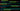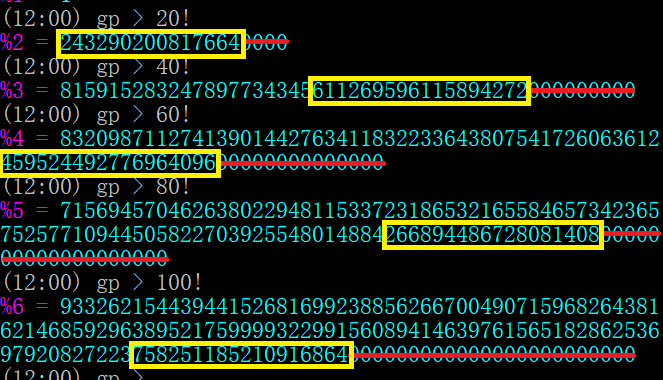## 计算阶乘的 18 位非零尾数

Fri, 22nd November 2019Edit on Githubfactorial阶乘

# 摘要

xstring于2005年在csdn挑战 ，对于一个给定的大整数，如何快速计算这个整数阶乘去掉尾零后的最低18位

gxqcn还利用他的HugeCalc 对算法重新实现并进行性能优化，比mathe用gmp 实现的代码更快了一个数量级。 贴子内容是mathe对https://blog.csdn.net/mathe/article/details/1132404 的备份。

# 详细内容

xstring首先发问: 阶乘不用解释吧，阶乘的 18 位非零尾数需要简单解释一下。阶乘的十进制结果中末尾都会有很多个数字 0 (5以下的除外)，去掉这些连续的 0 之后最后 18 位数字即是这里所说的 18 位非零尾数。之所以称这为“非零”，是因为通常最后一个数字是 0。举两个例子，10! = 3,628,800，其 18 位非零尾数为 36,288；24! = 620,448,401,733,239,439,360,000，其 18 位非零尾数为 044,840,173,323,943,936mathe给出了如下分析:

5的因子数目为: $[\frac{28}5]+[\frac{28}{25}]=6$

$O(L^3 \log(L)^2 + L^3 \log(L) \times T+T\times\log(T))$, 使用的空间复杂度为$O(L^3+L^2 \log(L)+T)$

ii)记$F(k,x)=(x\times 5^k+1)(x\times5^k+2)(x\times5^k+3)(x\times5^k+4)(x\times5^k+6)\dots(x\times5^k+5^k-1) (\mod 5^L)$ 其中连乘中所有5的倍数全部被去掉 那么$F(1,x)=24+50\times 5\times x+35\times 5^2\times x^2+10\times 5^3\times x^3+5^4\times x^4 (\mod 5^L)$.

$g(N,L) (\mod 5^L)$我们可以将它划分成若干个长度不一的类似ii)中的连乘式,其中长度不超过L的有L个，长度大于L的由于对应F(k,x)总是1,可以统一处理。对于每个长度不超过L的连乘式,我们代入公式ii)后, 需要L次乘法, 所以计算$g(N,L)(\mod 5^L)$共花费时间为$O(L^3 \log(L))$ v) 在计算完$g(N,L) (\mod 5^L)$以后,我们还需要计算$f([\frac{N}5],L)$,同样的,如果我们把N看成T位5进制数(事先转化一个数为5进制只需要$T\log(T)$的时间),那么$\frac{N}5$是T-1位5进制数,所以通过同样算法,可以再花费$O(L^3 \log(L))$的时间递归到T-2位数,...,这样总共经过T步后就会得出最终结果. 这个递归过程中总共花费时间最多为 $O(T\times L^3 \log(L))$ 而保存原式输入数据X (以及中间数据X/5, X/25,...等等)需要一个长度为T的空间,需要O(T)的空间 所以总共需要时间复杂度 $O(L^3 \log(L)^2 + L^3 \log(L) T + T \log(T))$ 使用的空间复杂度为$O(L^3+L^2 \log(L)+T)$

Github